# Resources tagged with: Similarity and congruence

Filter by: Content type:
Age range:
Challenge level:

There are 59 NRICH Mathematical resources connected to Similarity and congruence, you may find related items under Angles, Polygons, and Geometrical Proof.

Broad Topics > Angles, Polygons, and Geometrical Proof > Similarity and congruence### Fit for Photocopying

##### Age 14 to 16Challenge Level

Explore the relationships between different paper sizes.### Pythagoras Proofs

##### Age 14 to 16Challenge Level

Can you make sense of these three proofs of Pythagoras' Theorem?### Making Sixty

##### Age 14 to 16Challenge Level

Why does this fold create an angle of sixty degrees?### Partly Circles

##### Age 14 to 16Challenge Level

What is the same and what is different about these circle questions? What connections can you make?### Matching Triangles

##### Age 5 to 7Challenge Level

Can you sort these triangles into three different families and explain how you did it?### Nicely Similar

##### Age 14 to 16Challenge Level

If the hypotenuse (base) length is 100cm and if an extra line splits the base into 36cm and 64cm parts, what were the side lengths for the original right-angled triangle?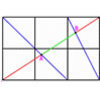##### Age 16 to 18Challenge Level

A new problem posed by Lyndon Baker who has devised many NRICH problems over the years.### Trapezium Four

##### Age 14 to 16Challenge Level

The diagonals of a trapezium divide it into four parts. Can you create a trapezium where three of those parts are equal in area?### Figure of Eight

##### Age 14 to 16Challenge Level

On a nine-point pegboard a band is stretched over 4 pegs in a "figure of 8" arrangement. How many different "figure of 8" arrangements can be made ?### Squirty

##### Age 14 to 16Challenge Level

Using a ruler, pencil and compasses only, it is possible to construct a square inside any triangle so that all four vertices touch the sides of the triangle.### Hex

##### Age 11 to 14Challenge Level

Explain how the thirteen pieces making up the regular hexagon shown in the diagram can be re-assembled to form three smaller regular hexagons congruent to each other.### Angle Trisection

##### Age 14 to 16Challenge Level

It is impossible to trisect an angle using only ruler and compasses but it can be done using a carpenter's square.### Napkin

##### Age 14 to 16Challenge Level

A napkin is folded so that a corner coincides with the midpoint of an opposite edge . Investigate the three triangles formed .### Sitting Pretty

##### Age 14 to 16Challenge Level

A circle of radius r touches two sides of a right angled triangle, sides x and y, and has its centre on the hypotenuse. Can you prove the formula linking x, y and r?##### Age 14 to 16Challenge Level

Two ladders are propped up against facing walls. The end of the first ladder is 10 metres above the foot of the first wall. The end of the second ladder is 5 metres above the foot of the second. . . .### Triangle Midpoints

##### Age 14 to 16Challenge Level

You are only given the three midpoints of the sides of a triangle. How can you construct the original triangle?### Doubly Isosceles

##### Age 14 to 16 ShortChallenge Level

Find the missing distance in this diagram with two isosceles triangles### Triangular Slope

##### Age 14 to 16 ShortChallenge Level

Can you find the gradients of the lines that form a triangle?### Another Triangle in a Triangle

##### Age 16 to 18Challenge Level

Can you work out the fraction of the original triangle that is covered by the green triangle?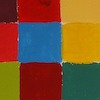### The Square Under the Hypotenuse

##### Age 14 to 16Challenge Level

Can you work out the side length of a square that just touches the hypotenuse of a right angled triangle?### Triangle in a Trapezium

##### Age 11 to 16Challenge Level

Can you find and prove the relationship between the area of a trapezium and the area of a triangle constructed within it?### Same Length

##### Age 11 to 16Challenge Level

Construct two equilateral triangles on a straight line. There are two lengths that look the same - can you prove it?### Kite in a Square

##### Age 14 to 16Challenge Level

Can you make sense of the three methods to work out the area of the kite in the square?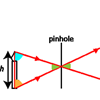### Pinhole Camera

##### Age 11 to 14Challenge Level

Make your own pinhole camera for safe observation of the sun, and find out how it works.##### Age 14 to 18Challenge Level

How would you design the tiering of seats in a stadium so that all spectators have a good view?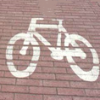### It Depends on Your Point of View!

##### Age 14 to 16Challenge Level

Anamorphic art is used to create intriguing illusions - can you work out how it is done?### Folding Fractions

##### Age 14 to 16Challenge Level

What fractions can you divide the diagonal of a square into by simple folding?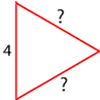### Number the Sides

##### Age 7 to 11Challenge Level

The triangles in these sets are similar - can you work out the lengths of the sides which have question marks?### Wedge on Wedge

##### Age 14 to 16Challenge Level

Two right-angled triangles are connected together as part of a structure. An object is dropped from the top of the green triangle where does it pass the base of the blue triangle?### Points in Pairs

##### Age 14 to 16Challenge Level

Move the point P to see how P' moves. Then use your insights to calculate a missing length.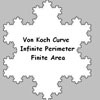### Von Koch Curve

##### Age 16 to 18Challenge Level

Make a poster using equilateral triangles with sides 27, 9, 3 and 1 units assembled as stage 3 of the Von Koch fractal. Investigate areas & lengths when you repeat a process infinitely often.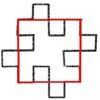### Squareflake

##### Age 16 to 18Challenge Level

A finite area inside and infinite skin! You can paint the interior of this fractal with a small tin of paint but you could never get enough paint to paint the edge.### Sierpinski Triangle

##### Age 16 to 18Challenge Level

What is the total area of the triangles remaining in the nth stage of constructing a Sierpinski Triangle? Work out the dimension of this fractal.### Triangle in a Triangle

##### Age 14 to 16Challenge Level

Can you work out the fraction of the original triangle that is covered by the inner triangle?### Pentabuild

##### Age 16 to 18Challenge Level

Explain how to construct a regular pentagon accurately using a straight edge and compass.### Slippage

##### Age 14 to 16Challenge Level

A ladder 3m long rests against a wall with one end a short distance from its base. Between the wall and the base of a ladder is a garden storage box 1m tall and 1m high. What is the maximum distance. . . .### Bus Stop

##### Age 14 to 16Challenge Level

Two buses leave at the same time from two towns Shipton and Veston on the same long road, travelling towards each other. At each mile along the road are milestones. The buses' speeds are constant. . . .### All Tied Up

##### Age 14 to 16Challenge Level

A ribbon runs around a box so that it makes a complete loop with two parallel pieces of ribbon on the top. How long will the ribbon be?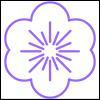### Flower

##### Age 16 to 18Challenge Level

Six circles around a central circle make a flower. Watch the flower as you change the radii in this circle packing. Prove that with the given ratios of the radii the petals touch and fit perfectly.### Arrh!

##### Age 14 to 16Challenge Level

Triangle ABC is equilateral. D, the midpoint of BC, is the centre of the semi-circle whose radius is R which touches AB and AC, as well as a smaller circle with radius r which also touches AB and AC. . . .### Two Triangles in a Square

##### Age 14 to 16Challenge Level

Given that ABCD is a square, M is the mid point of AD and CP is perpendicular to MB with P on MB, prove DP = DC.##### Age 14 to 16Challenge Level

Find the area of the shaded region created by the two overlapping triangles in terms of a and b?### Pentakite

##### Age 14 to 18Challenge Level

ABCDE is a regular pentagon of side length one unit. BC produced meets ED produced at F. Show that triangle CDF is congruent to triangle EDB. Find the length of BE.### Chord

##### Age 16 to 18Challenge Level

Equal touching circles have centres on a line. From a point of this line on a circle, a tangent is drawn to the farthest circle. Find the lengths of chords where the line cuts the other circles.### Overlap

##### Age 11 to 14Challenge Level

A red square and a blue square overlap so that the corner of the red square rests on the centre of the blue square. Show that, whatever the orientation of the red square, it covers a quarter of the. . . .### Triangular Tantaliser

##### Age 11 to 14Challenge Level

Draw all the possible distinct triangles on a 4 x 4 dotty grid. Convince me that you have all possible triangles.### Parallel Universe

##### Age 14 to 16Challenge Level

An equilateral triangle is constructed on BC. A line QD is drawn, where Q is the midpoint of AC. Prove that AB // QD.### Matter of Scale

##### Age 14 to 16Challenge Level

Prove Pythagoras' Theorem using enlargements and scale factors.### Pent

##### Age 14 to 18Challenge Level

The diagram shows a regular pentagon with sides of unit length. Find all the angles in the diagram. Prove that the quadrilateral shown in red is a rhombus.### Folding Squares

##### Age 14 to 16Challenge Level

The diagonal of a square intersects the line joining one of the unused corners to the midpoint of the opposite side. What do you notice about the line segments produced?